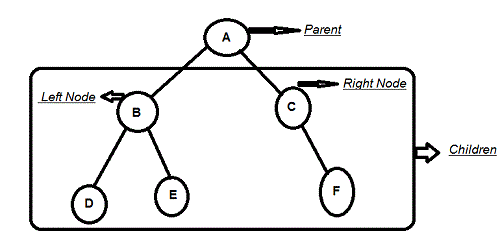# Binary Tree Assignment Help

## Binary TreeThe tree data structure which has at most two children is called Binary tree. It is made of nodes, which each node contains a “left” reference, a “right” reference and a data element. The topmost node in the tree is called “root”. Every node except a root in a tree is connected by a directed edge from exactly one other node. This is known as parent.

And on the other hand each node can be connected to arbitrary number of nodes, called children. The nodes which does not have any children are called leaves, or external nodes. Nodes which are not leaves are called internal nodes. Nodes which are connected to same parent are called sibling.

A binary tree is a tree data structures in which each node have at most two child nodes, usually identify as left and right. Nodes with children are parent nodes, and child nodes may contain references to their parents.

For example binary tree can have two children i.e. called left and right child and one can leap from one node to another on a binary tree, in three contrary ways- preorder, inorder and postorder transversals.

### More tree terminology:

• The height of the tree is the height of the root.
• A full binary tree is a binary tree in which each node has exactly zero or two children.
• A complete binary tree is a binary tree, which is completely filled.
• The depth of a node is the number of edges from the root to the node.### Types of binary trees

1. Rooted Binary Tree

It is with a root node in which every node has at most two children.

2. Full Binary Tree

It is also known as proper binary tree. In this type of tree every node has two children other than the leaves.

3. Perfect Binary Tree

It is similar to full binary tree in which all leaves have same depth or same level and in this every parent has two children.

4. Complete Binary Tree

In this type of binary tree every level, except possibly the last, is completely filled, and all nodes are as far as possible.

5. Infinite Complete Binary Tree

In this type of binary tree in which there are countable infinite number of levels, in which every node has two children, so that there are 2d nodes at level d.

6. Balanced Binary Tree

It is defined as binary tree in which the depth off the left and right sub trees of every node differ by 1 or less.

7. Degenerate Tree

It is also called Pathological tree. In this type of tree each parent node has only one associated child node.Find the best Binary Tree Assignment Help Services with us

Try our determination care now, solution of your problem is righteous a depression departed. Knock any quantify at our 24x7 live supports for any ask. To know about how to proceed, just visit how it Works page at Assignmenthelp.net